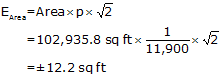## 5. Accuracy Revisited

### a. How Accurate?

Which method, Coordinates or DMDs, is most accurate? Because both area calculation methods use the same adjusted latitudes and departures, they should have equal accuracy. The best way to determine area accuracy is to propagate all measurement and setup errors into it. Even with a computer that can be a daunting task. If surveying software is available, a sensitivity analysis can be done: vary multiple measurements by introducing reasonable errors into them and examine how the area changes.

A simplified approach can be used which uses basic error propagation along with the traverse precision. Envision a rectangular parcel which includes the same area as the traverse. Because the area of a rectangle is length times width, the Error of a Product can be used to approximate an accuracy, Figure G-14.Figure G-14 Error of a Product

The equation for Error of a Product is:Equation G-7

in terms of the rectangle:Equation G-8

EW and EL are the expected errors in the width and length. What do we use for EW and EL?  We can approximate the errors using the traverse precision. The precision summarizes up the overall random error effects for that particular traverse. If we multiply a distance by the precision, p, it gives us an expected error in that distance.

 EL = L x p EW = W x p Equations G-9 and G-10

Substituting that back into the equation and reducing:Equation G-11

This simplified method takes into account the overall quality of the traverse in the form of its precision along with the magnitude of its size.

### b. Examples

#### (1) Bearing Traverse, Figure G-11

Prior to adjustment we determined its precision to be 1/11,900; area computed by coordinates is 102,935.8 sq ft.

Substituting those numbers into Equation G-11:Recall that throughout our calculations, up to that of the area, we carried an additional digit to minimize rounding errors. That means our area uncertainty would be expressed to two sig fig: ±12 sq ft.

The area should be expressed to a level compatible with the uncertainty: 102,936 sq ft.

#### (2) Crossing Traverse Parcel Survey

Prior to adjustment we determined the Crossing Traverse precision to be 1/10,100. After adjustment its coordinates were used to compute the Parcel's area as 90,018.76 sq ft.

Substituting those numbers into Equation G-11:The Parcel's area uncertainty is ±12 sq ft so show it as 90,018 sq ft.It happens because of their combination of area and precision that both examples have ±12 sq ft error. Don't assume all areas will have the same error.

### c. A Final Word

We generally have a tendency to overstate area accuracy: it's not uncommon to see a computer generated map with areas expressed to 0.01 sq ft. Surveyors sometimes get caught up in equipment specifications and forget about the propagated errors when multiple measurements are combined. After all, our total station reports distances to 0.01 ft and angles to 01". That should result in an uncertainty smaller than 12 sq ft, shouldn't it?

The area of the square in Figure G-15 on your screen should be 0.01 sq ft; it is 0.1 ft (approx 1.2") on a side (Your browser's magnification and display may affect the square's displayed size. If that's the case, draw a 1.2" square on a piece of paper to visualize it.).Figure G-15 Area Resolution?

Knowing what we do about how errors occur and accumulate, does it make sense to state an area of 90,018 sq ft to an accuracy of that square?

Consider this: How much would the area of the Bearing Traverse example be affected if we offset line AB by 0.01 ft?Figure G-16 Slightly Displacing a Line

We could approximate the area change with a 0.01' wide by 472.72 ft long rectangle - a difference of about 4.7 sq ft. That 0.01 ft offset would represent relatively small random errors in both lines CB and DA. Instrument and reflector centering errors and measurement errors could easily exceed 0.01 ft error per line. Include pointing errors measuring angles and the potential error can be substantial. Using similar equipment and procedures measuring larger traverses or traverses with more points will also increase the error in the area. So an error of 12 sq ft doesn't seem so bad now, does it?

Using the simplified error propagation, Equation G-10 allows the surveyor to express area to a reasonable level of uncertainty.